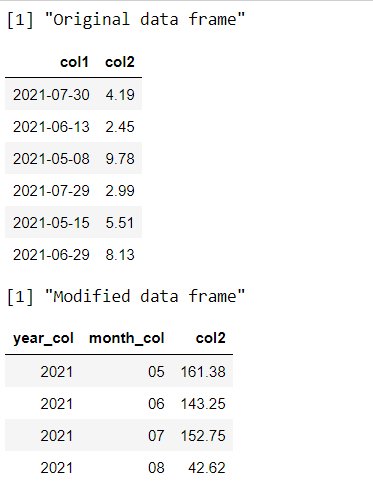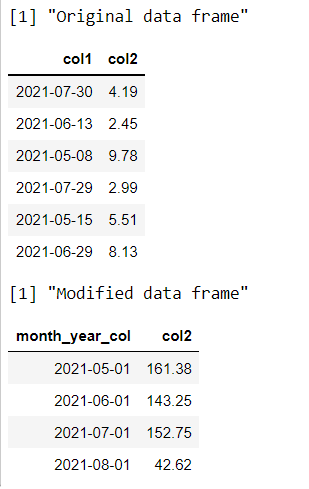Related Articles

# Aggregate Daily Data to Month and Year Intervals in R DataFrame

• Last Updated : 23 Sep, 2021

In this article, we are going to see how to aggregate daily data over a period of months and year intervals in dataframe in R Programming Language.

## Method 1 : Using aggregate() method

Base R contains a large number of methods to perform operations on the dataframe. The seq() method in R is used to generate regular sequences beginning from a pre-defined value.

Syntax: seq(from , to , by , length.out)

Arguments :

• from – The value from where to begin the sequence. The as.Date() method is used here, in order to generate a sequence of dates until the length of the sequence is met.
• to – The value where to end the sequence.
• by – The parameter to increment the sequence. “day” is used as a parameter here, in order to generate successive dates in order.
• length.out – The total length of the sequence.

The dataframe is then formed using a sample from this date sequence generated as column 1. The value is generated using the rnorm() method to produce random floating-point numbers.

The strftime() method is then used to convert a time object to a character string back. The format can be specified to extract different components of the date object.

Syntax: strftime (date, format)

Arguments :

• date – The object to be converted
• format – We use %m to extract the month and %Y to extract the year in YYYY format.

In order to aggregate the data, the aggregate method is used, which is used to compute summary statistics of each of the groups.

Syntax: aggregate ( formula , data , FUN)

Arguments :

• formula – a formula, such as y ~ x
• data – The dataframe over which to apply the function
• FUN – The function to be applied to the dataframe.  Here, the function applied is sum in order to perform the aggregation or summation over the values belonging to same group.

Code:

## R

 `set.seed``(99923) `` ` `# creating dataframe``# specifying number of rows``len <- 100`` ` `# creating sequences of dates``var_seq <- ``seq``(``as.Date``(``"2021/05/01"``),``               ``by = ``"day"``,``               ``length.out = len)`` ` `# creating columns for dataframe``data_frame <- ``data.frame``(col1 = ``sample``( var_seq,``                                 ``100, replace = ``TRUE``),``                   ``col2 = ``round``(``rnorm``(10, 5, 2), 2))``print``(``"Original dataframe"``)``head``(data_frame)`` ` `# creating new year column for dataframe``data_frame\$year_col <- ``strftime``(data_frame\$col1, ``"%Y"``)   `` ` `# creating new month column for dataframe``data_frame\$month_col <- ``strftime``(data_frame\$col1, ``"%m"``)`` ` `# aggregating the daily data``data_frame_mod <- ``aggregate``(col2 ~ year_col + month_col,       ``                        ``data_frame,``                        ``FUN = sum)``print``(``"Modified dataframe"``)``head``(data_frame_mod)`

Output:## Method 2: Using lubridate and dplyr package

Lubridate package in R is used to provide easier working mechanisms with the date and time objects. It can be loaded and installed in the working space using the following command :

`install.packages("lubridate")`

The floor_date() method in R uses a date-time object, may be a single entity or vector of date-time objects, and then further rounds it off to the nearest integer value in the specified unit of time.

`floor_date(x , unit = months)`

The dplyr package is used to perform data manipulations and statistics. It can be loaded and installed in the working space using the following command :

`install.packages("dplyr")`

The dataframe is modified by using the piping operator over a sequence of operations and methods. The group_by() method is used to group the data based on the values contained in the specified columns.

`group_by(col1,..)`

Then a summary statistic is performed using the summarize() method which performs a summation over the values contained in the third column. The result is then manipulated into a dataframe using the as.data.frame() method.

Code:

## R

 `library``(``"dplyr"``)``library``(``"lubridate"``)``set.seed``(99923) `` ` `# creating dataframe``# specifying number of rows``len <- 100`` ` `# creating sequences of dates``var_seq <- ``seq``(``as.Date``(``"2021/05/01"``),``               ``by = ``"day"``,``               ``length.out = len)`` ` `# creating columns for dataframe``data_frame <- ``data.frame``(col1 = ``sample``( var_seq,``                                 ``100, replace = ``TRUE``),``                   ``col2 = ``round``(``rnorm``(10, 5, 2), 2))``print``(``"Original dataframe"``)``head``(data_frame)`` ` `# creating new month column for dataframe``data_frame\$month_year_col <- ``floor_date``(data_frame\$col1, ``                                   ``"month"``)``# aggregating the daily data``data_frame_mod <- data_frame %>%                        ``  ``group_by``(month_year_col) %>% ``  ``dplyr::``summarize``(col2 = ``sum``(col2)) %>% ``  ``as.data.frame``()`` ` `print``(``"Modified dataframe"``)``head``(data_frame_mod)`

Output:My Personal Notes arrow_drop_up